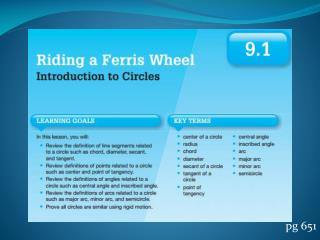DownloadDownload Presentationpg 651

# pg 651

Télécharger la présentation## pg 651

- - - - - - - - - - - - - - - - - - - - - - - - - - - E N D - - - - - - - - - - - - - - - - - - - - - - - - - - -
##### Presentation Transcript

1. pg 651

2. A chord is a line segment with each endpoint on the circle A diameter is a chord that passes through the center of the circle. A secant of a circle is a line that intersects a circle at exactly two points 1. Draw a chord 2. Draw a diameter 3. Draw a secant

3. A tangent of a circle is a line that intersects a circle at exactly one point. The point of intersection is called the point of tangency. A central angle is an angle whose vertex is the center of the circle. An inscribed angle is an angle whose vertex is on the circle. Draw a line tangent to circle O with a point of tangency at Wesley.

4. pg 656

5. pg 660

6. pg 664

7. Pg 666

8. pg672

9. pg674